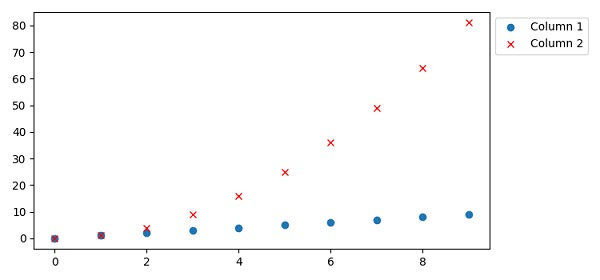# How to put a legend outside the plot with Pandas?

PandasPythonServer Side ProgrammingProgramming

To put a legend outside the plot with Pandas, we can take the following Steps −

• Make a dictionary d with keys Column1 and Column2.

• Make a data frame using DataFrame (d).

• Plot the data frame with a list of styles.

• Using legend(), place a legend on the figure. The bbox_to_anchor keyword gives a great degree of control for manual legend placement. For example, if you want your axes legend located at the figure's top right-hand corner instead of the axes' corner, simply specify the corner's location, and the coordinate system of that location.

• To display the figure, use the show() method.

## Example

import pandas as pd
from matplotlib import pyplot as plt
plt.rcParams["figure.figsize"] = [7.50, 3.50]
plt.rcParams["figure.autolayout"] = True
d = {'Column 1': [i for i in range(10)],
'Column 2': [i * i for i in range(10)]}
df = pd.DataFrame(d)
df.plot(style=['o', 'rx'])
plt.legend(bbox_to_anchor=(1.0, 1.0))
plt.show()

## Output